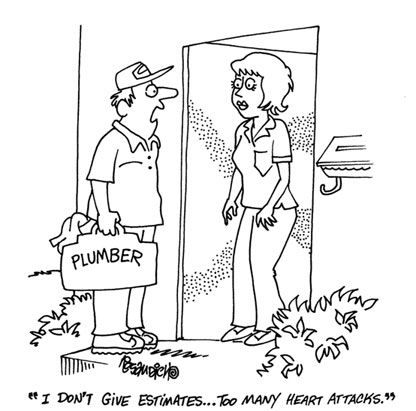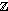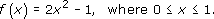Chambers English Dictionary defines the word data as follows.

data, dātä,, n.pl. facts given, from which others may be inferred:—sing. da'tum(q.v.) …. [L. data, things given, pa.p. neut. pl. of dare, to give.]

You might prefer the definition given in the Shorter Oxford English Dictionary.

data, things given or granted; something known or assumed as fact, and made the basis of reasoning or calculation.

Author(s): The Open University## Study another free course

There are more than 800 courses on OpenLearn for you to
Author(s): The Open University

Now try the quiz and see if there are any areas you need to work on.

Author(s): The Open University

## Activity 33

The population of a village is 5481. Round this:

• (a) to the nearest thousand people;

• (b) to the nearest hundred people.

Author(s): The Open University

## Activity 27

Give the appropriate rounding for each of the values below:

• (a) Carpet floor area = 26.456 sq metres

• (b) Interest earned = £109.876 5439

• Author(s): The Open UniversityWhen using a calculator many people have ‘blind
Author(s): The Open University

## Activity 21

Without using your calculator solve the following calculations.

• (a) 3 + 5 × 2 = ?

• (b) 12 − 6 + 6 = ?

• (c) 6 + (5 +
Author(s): The Open University

You can use the same procedure for numbers less than one.

## Example 4

In scientific work people deal with very small units of measurement. Suppose you read that the spacing between adjacent atoms in a solid was 0.000 002 456 84 metres. You could make the number more memorable
Author(s): The Open University

After studying this course, you should be able to:

• round a given whole number to the nearest 10, 100, 1000 and so on

• round a decimal number to a given number of decimal places or significant figures

• use rounded numbers to find rough estimates for calculations

• use a calculator for decimal calculations involving +, −, × and ÷, giving the answer to a specified accuracy (e.g. decimal places or significant figures) and checking the ans
Author(s): The Open University

• 1.   The condition ‘is equal to’ is a relation on any set of real numbers because, for any x, y in the set, the statement ‘x is equal to y’ is either definitely true or definitely false. This relation is usually denoted by the symbol =. For this relation, each real number in the set is related only to itself!

• 2.   The condition ‘is less than’ is a relation on any set of real numbers, and we usually
Author(s): The Open University

In the last subsection we stated that, for any integer n ≥ 2, the setn satisfies the same rules for addition modulo n as the real numbers satisfy for ordinary addition. When it comes to multiplicat
Author(s): The Open University

You have seen that the complex number x + iy corresponds to the point (x, y) in the complex plane. This correspondence enables us to give an alternative description of complex numbers, using so-called polar form. This form is particularly useful when we discuss properties related to multiplication and division of complex numbers.

[Image_Link]https://www.open.edu/openlearn/ocw/pluginfile.php/89651/mod_oucontent/ouconte
Author(s):
The Open University

Arithmetical operations on complex numbers are carried out as for real numbers, except that we replace i2 by −1 wherever it occurs.

## Example 1

Let z1 = 1 + 2i and z<
Author(s): The Open University

In OpenLearn course Diagrams, graphs and charts you saw how a scale is used on plans of houses and other structures. The scale makes it possible to take a length on the plan and calculate the correspond
Author(s): The Open University

What is a volume? The word usually refers to the amount of three-dimensional space that an object occupies. It is commonly measured in cubic centimetres (cm3) or cubic metres (m3).

A closely related idea is capacity; this is used to specify the volume of liquid or gas that a container can actually hold. You might refer to the volume of a brick and the capacity of a jug – but not vice versa. Note that a container with a particular volume will not nec
Author(s): The Open University

Look at the shapes below. The symmetry of the shape on the left and its relationship to the shape on the right can be thought of in two ways:

• Fold the left-hand shape along the central line. Then one side lies exactly on top of the other, and gives the shape on the right.

• Imagine a mirror placed along the central dotted line. The reflection in the mirror gives the other half of the shape.

Author(s): The Open University

## Example 14

Suppose you have decided to tile the wall using square tiles of side 10 cm. You are proposing to use the tiles across the full 5 metre width of the wall up to a height of 1.8 m.

Find the number of boxes of tiles that you will require to cover the wall if the tiles a
Author(s): The Open University

In this type of question you are given the answer! All the marks are allocated for correct reasoning and justification.

## Example 15

Suppose you now decide to place your new bath (length 1.7 m, height 0.8 m) against this wall as shown in the diagram below.

Author(s): The Open University

A fundamental concept in mathematics is that of a function.

Consider, for example, the function f defined byThis is an example of a real function, because it associates with a given real number
Author(s): The Open University

World in transition: Migration and Trade
What does it mean to be poor, or an immigrant? What form should Aid take? This album begins to explore the complex issues of international development in a globalised world, starting with a look at schemes which attempt to alleviate poverty. Small business owners are empowered by micro-financing in Glasgow, while in Argentina a disastrous economic collapse has led to people taking matters into their own hands by creating an alternative social exchange currency. Migrant communities, such as Greek
Author(s): The OpenLearn team